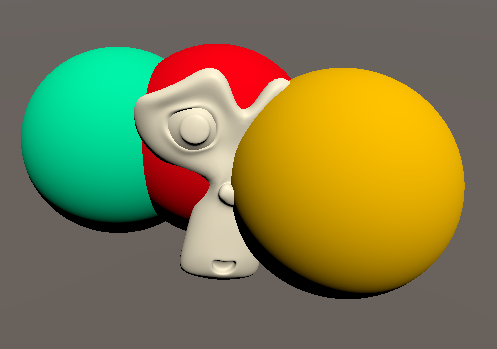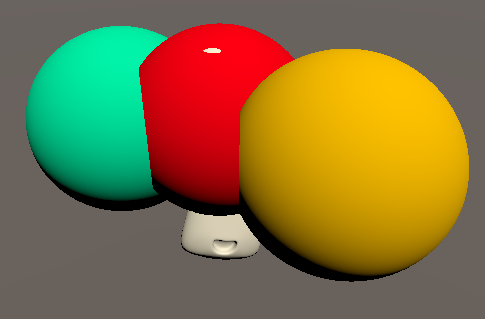In the last 2 tutorials of the volumetric rendering series I showed how to trace 3d signed distance fields and how to shade the result. In my opinion the biggest drawback of the state of the shader so far is the way that independent objects interact with each other and with regular meshes. They either don’t write to the depth buffer at all, or with the shape of the mesh that’s used for them and the depth check is similarly lacking. In this tutorial I want to show you how to make volumetric rendering work with the depth buffer just as you expect it to.

This tutorial starts with the code of the shaded spheretracing tutorial and you should at least understand spheretracing basics before you try to understand it.If you followed my previous tutorials on volumetric rendering tutorials I want to change a few small things for this one. Since we’re fixing the depth, we can now enable the depth writing for the shader by adding `ZWrite On` to the SubShader or Pass section. I also set the Render Type to Opaque as well as define the render queue to render the object just after regular geometry in the Tags of the SubShader.

``````//the material is completely non-transparent and is rendered just after opaque geometry
Tags{ "RenderType"="Opaque" "Queue"="Geometry+1" "DisableBatching"="True" "IgnoreProjector"="True"}
``````

Additionally I want to change the setup of the tracing loop since the color of the material is decided in a function call inside a conditional(if) statement in the loop. I’m not 100% sure what operations affect performance in which ways, but my gut feeling tells me that it’s more efficient to do the heavy operations outside of the loop. And keep calculations in conditional blocks as lightweight as possible. I archived this by defining a `hitsurface` variable before the loop starts and set it to false and if we actually hit a surface, it’s set to true and the loop is aborted. This way we can then discard all pixels which rays didn’t result in a surface hit after the loop and then return the color. Another small change was that I renamed the function which calculates the color to `renderSurface` and defined the samplePoint variable before the loop so we still have access to it after it finished. With this the new fragment function should look like this:

``````fixed4 frag(v2f i){
//ray information
float3 pos = i.localPosition;
float3 dir = normalize(i.viewDirection.xyz);
float progress = 0;
float3 samplePoint = 0;

bool hitsurface = false;
//tracing loop
for (uint iter = 0; iter < MAX_STEPS; iter++) {
//get current location on ray
samplePoint = pos + dir * progress;
//get distance to closest shape
float distance = scene(samplePoint);
//return color if inside shape
if(distance < THICKNESS){
hitsurface = true;
break;
}
//go forwards
progress = progress + distance;
}
//discard pixel if no shape was hit
clip(hitsurface ? 1 : -1);

//return surface color
return renderSurface(samplePoint);
}
``````## Output Custom Depth

If we don’t worry about the depth of our surface, the shader pipeline automatically uses the depth of the triangles of the mesh. But we also have the possibility on many platforms to write and compare whatever depth value we want to. To write custom depth values we can either return a struct from the fragment function with variables for both color and depth or what I opted to do, remove the output type of the function by changing it to void and instead define output variables for the color and depth values.

``````void frag(v2f i, out fixed4 color : SV_TARGET, out float depth : SV_Depth){
``````

The color variable we can set to the value we returned previously. For the depth value we have to calculate the distance to the camera, the most forward way to do that is to get it from the clip space position. We calculate the clip space position in a similar way we transform vertices into clip space in the vertex shader, via the `UnityObjectToClipPos` macro. The `float4` result of this also has the `w` component which we have to divide by to get regular 3d values. Since we only care about the depth, we only divide the `z` component by the `w` component and assign the result to the depth output value. Because we changed the function type to void we don’t have to return anything.

``````//calculate surface color
color = renderSurface(samplePoint);
//calculate surface depth
float4 tracedClipPos = UnityObjectToClipPos(float4(samplePoint, 1.0));
depth = tracedClipPos.z / tracedClipPos.w;
``````## Source

``````Shader "Tutorial/045_SphereTracingDepth"{
//show values to edit in inspector
Properties{
_Color ("Color", Color) = (0, 0, 0, 1)
}

//the material is completely non-transparent and is rendered just after opaque geometry
Tags{ "RenderType"="Opaque" "Queue"="Geometry+1" "DisableBatching"="True" "IgnoreProjector"="True"}

Pass{
ZWrite On

CGPROGRAM
#include "UnityCG.cginc"
#include "Lighting.cginc"

#pragma vertex vert
#pragma fragment frag

//surface color
fixed4 _Color;

//maximum amount of steps
#define MAX_STEPS 32
//furthest distance that's accepted as inside surface
#define THICKNESS 0.001
//distance from rendered point to sample SDF for normal calculation
#define NORMAL_EPSILON 0.01

//input data
struct appdata{
float4 vertex : POSITION;
};

//data that goes from vertex to fragment shader
struct v2f{
float4 position : SV_POSITION; //position in clip space
float4 localPosition : TEXCOORD0; //position in local space
float4 viewDirection : TEXCOORD1; //view direction in local space (not normalized!)
};

v2f vert(appdata v){
v2f o;
//position for rendering
o.position = UnityObjectToClipPos(v.vertex);
//save local position for origin
o.localPosition = v.vertex;
//get camera position in local space
float4 objectSpaceCameraPos = mul(unity_WorldToObject, float4(_WorldSpaceCameraPos, 1));
//get local view vector
o.viewDirection = v.vertex - objectSpaceCameraPos;
return o;
}

float scene(float3 pos){
return length(pos) - 0.5;
}

float3 normal(float3 pos){
//determine change in signed distance
float changeX = scene(pos + float3(NORMAL_EPSILON, 0, 0)) - scene(pos - float3(NORMAL_EPSILON, 0, 0));
float changeY = scene(pos + float3(0, NORMAL_EPSILON, 0)) - scene(pos - float3(0, NORMAL_EPSILON, 0));
float changeZ = scene(pos + float3(0, 0, NORMAL_EPSILON)) - scene(pos - float3(0, 0, NORMAL_EPSILON));
//construct normal vector
float3 surfaceNormal = float3(changeX, changeY, changeZ);
//convert normal vector into worldspace and make it uniform length
surfaceNormal = mul(unity_ObjectToWorld, float4(surfaceNormal, 0));
return normalize(surfaceNormal);
}

float4 lightColor(float3 position){
//calculate needed surface and light data
float3 surfaceNormal = normal(position);
float3 lightDirection = _WorldSpaceLightPos0.xyz;

float lightAngle = saturate(dot(surfaceNormal, lightDirection));
return lightAngle * _LightColor0;
}

float4 renderSurface(float3 position){
//get light color
float4 light = lightColor(position);

//combine base color and light color
float4 color = _Color * light;

return color;
}

void frag(v2f i, out fixed4 color : SV_TARGET, out float depth : SV_Depth){
//ray information
float3 pos = i.localPosition;
float3 dir = normalize(i.viewDirection.xyz);
float progress = 0;
float3 samplePoint = 0;

bool hitsurface = false;
//tracing loop
for (uint iter = 0; iter < MAX_STEPS; iter++) {
//get current location on ray
samplePoint = pos + dir * progress;
//get distance to closest shape
float distance = scene(samplePoint);
//return color if inside shape
if(distance < THICKNESS){
hitsurface = true;
break;
}
//go forwards
progress = progress + distance;
}
//discard pixel if no shape was hit
clip(hitsurface ? 1 : -1);

//calculate surface color
color = renderSurface(samplePoint);
//calculate surface depth
float4 tracedClipPos = UnityObjectToClipPos(float4(samplePoint, 1.0));
depth = tracedClipPos.z / tracedClipPos.w;
}

ENDCG
}
}
}
``````

You can also find me on twitter at @totallyRonja. If you liked my tutorial and want to support me you can do that on Patreon (patreon.com/RonjaTutorials) or Ko-Fi (ko-fi.com/RonjaTutorials).